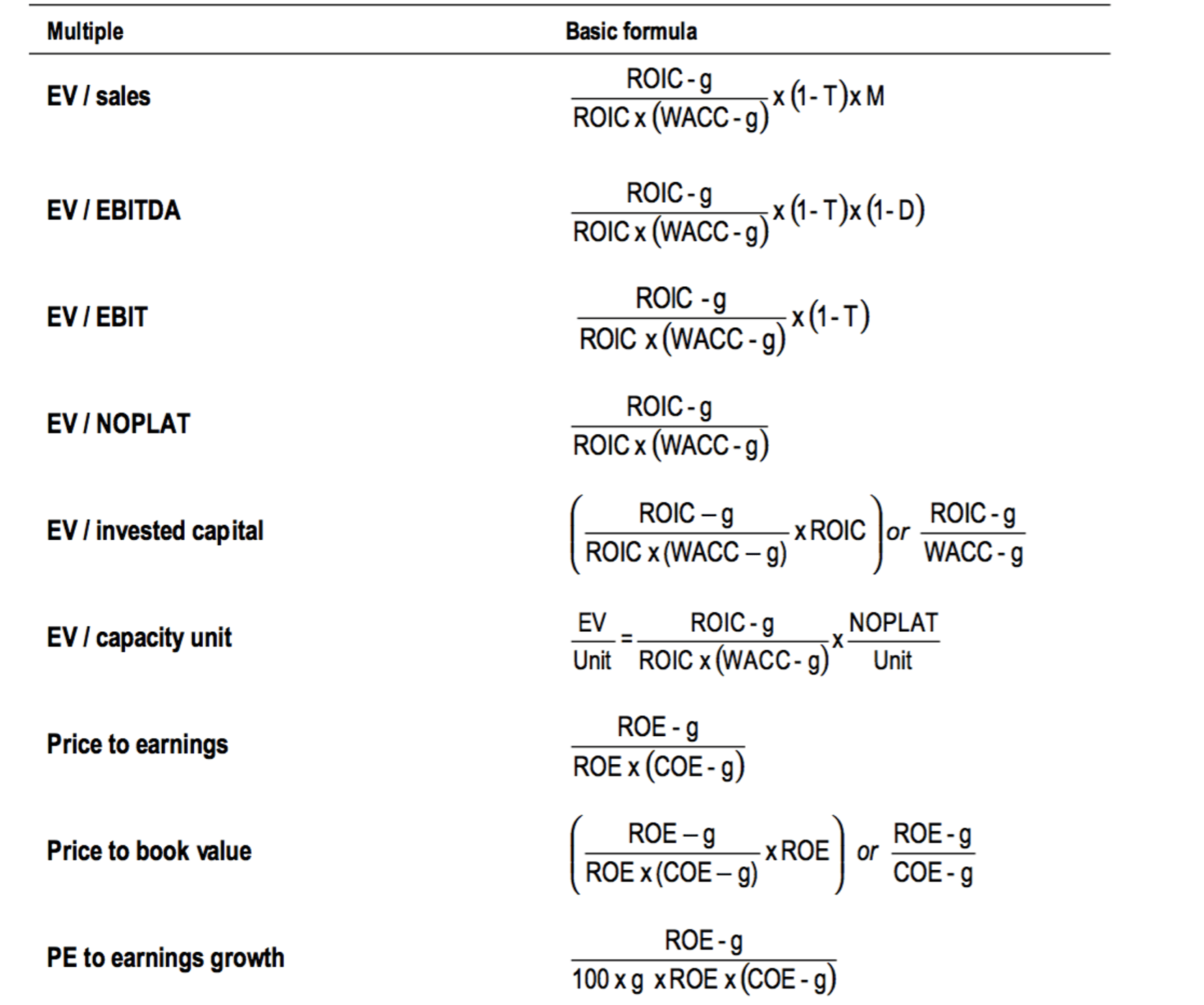# Target multiplesNote:

ROIC and ROE = incremental post-tax return on incremental total investment and equity

g = assumed

long-term growth rate

WACC = weighted average cost of capital

COE = cost of equity

T = effective tax rate

D = depreciation and amortisation as a % of EBITDA; M = operating margin

Word of caution:

These are based on limiting assumptions and should be used with care. For example, margins, depreciation, taxation and rate of investment are all assumed to remain constant, and growth is assumed to continue into perpetuity. As a result, multiples obtained using these formulas are likely to over- or understate fair value.

TAG:金融 | 投资 | 投资银行 |